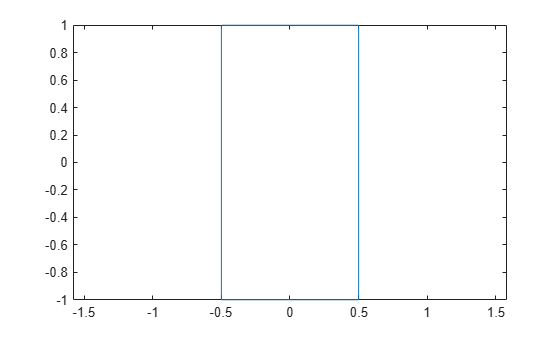plot

Plot boundary of shape

Description

example

p = plot(shape,varargin) plots the boundary of the shape and returns the line.

Examples

collapse all

Create a rectangular shape and plot it.

r = antenna.Rectangle;
p = plot(r);Input Arguments

collapse all

Shape created using custom elements and shape objects of Antenna Toolbox, specified as an object.

Example: plot(rectangle) where rectangle is created using antenna.Rectangle object.